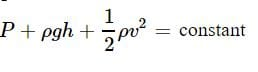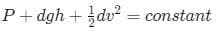NEET  >  Test: Applications of Bernoulli’s Principle

# Test: Applications of Bernoulli’s Principle

Test Description

## 10 Questions MCQ Test Physics Class 11 | Test: Applications of Bernoulli’s Principle

Test: Applications of Bernoulli’s Principle for NEET 2023 is part of Physics Class 11 preparation. The Test: Applications of Bernoulli’s Principle questions and answers have been prepared according to the NEET exam syllabus.The Test: Applications of Bernoulli’s Principle MCQs are made for NEET 2023 Exam. Find important definitions, questions, notes, meanings, examples, exercises, MCQs and online tests for Test: Applications of Bernoulli’s Principle below.
Solutions of Test: Applications of Bernoulli’s Principle questions in English are available as part of our Physics Class 11 for NEET & Test: Applications of Bernoulli’s Principle solutions in Hindi for Physics Class 11 course. Download more important topics, notes, lectures and mock test series for NEET Exam by signing up for free. Attempt Test: Applications of Bernoulli’s Principle | 10 questions in 10 minutes | Mock test for NEET preparation | Free important questions MCQ to study Physics Class 11 for NEET Exam | Download free PDF with solutions
 1 Crore+ students have signed up on EduRev. Have you?
Test: Applications of Bernoulli’s Principle - Question 1

### Bernoulli’s theorem includes as a special case of:

Detailed Solution for Test: Applications of Bernoulli’s Principle - Question 1

Torricelli's law states that the speed of efflux, v of a fluid through a sharp-edged hole at the bottom of a tank filled to a depth h is the same as the speed that a body (in this case a drop of water) would acquire in falling freely from a height h.
This problem is solved using Bernoulli's equation,
gh+Patm/ρ​​=v2/2​+Patm
⟹v=√2gh​

Test: Applications of Bernoulli’s Principle - Question 2

### Bernoulli’s theorem is important in the field of:

Detailed Solution for Test: Applications of Bernoulli’s Principle - Question 2

The Bernoulli equation is an important expression relating pressure, height and velocity of a fluid at one point along its flow.

Test: Applications of Bernoulli’s Principle - Question 3

### Water is flowing through a horizontal tube. The pressure of the liquid in the portion where velocity is 2 m/s is 2 m of Hg. What will be the pressure in the portion where velocity is 4 m/s?

Detailed Solution for Test: Applications of Bernoulli’s Principle - Question 3Test: Applications of Bernoulli’s Principle - Question 4

Air is streaming past a horizontal airplane wing such that its speed is 120 m/s over the upper surface and 90 m/s at the lower surface. If the density of the air is 1.3 kg/m3and the wing is 10 m long and has an average width of 2 m, then the difference of pressure on the two sides of the wing is

Detailed Solution for Test: Applications of Bernoulli’s Principle - Question 4

Applying Bernoulli's principle, we have
P1+1/2​ρv12=P2+1/2​ρv22
⇒P2​−P1=1/2ρ (v2/1−v2/2)
⇒ΔP=1/2​×1.3× (1202−902)
⇒ΔP=0.65× (120+90) × (120−90)
⇒ΔP=0.65×210×30=4095Pa

Test: Applications of Bernoulli’s Principle - Question 5

In which of the following types of flows is the Bernoulli’s theorem strictly applicable:

Detailed Solution for Test: Applications of Bernoulli’s Principle - Question 5

Bernoulli's principle is applicable on those non-viscous liquids which have laminar flow or streamlined flow.
It means that a liquid in which its particles exert no force on each other, i.e., the speed of all the particles of the liquid is same.
Also, the liquid should travel in the form of streamlines, i.e., the liquid flowing in one pipeline (imaginary pipeline) should not mix in the liquid in another pipeline. This is called streamlined flow.
Also, turbulent flow is the opposite of streamlined flow. So, turbulent liquid will not obey Bernoulli's principle.
But if the liquid is rotated, this streamlined flow will not take place. Thus Bernoulli's principle will not be applicable to this type of liquid.

Test: Applications of Bernoulli’s Principle - Question 6

In the houses far away from the municipal water tanks often people find it difficult to get water on the top floor. This happens because

Detailed Solution for Test: Applications of Bernoulli’s Principle - Question 6

In the houses far away from the municipal water tanks often people find it difficult to get water on the top floor. This happens because a lot of water pressure is lost as friction between water and the pipeline.

Test: Applications of Bernoulli’s Principle - Question 7

Water is flowing through a pipe under constant pressure. At some place the pipe becomes narrow. The pressure of water at this place:

Detailed Solution for Test: Applications of Bernoulli’s Principle - Question 7

Given, That area of cross section at initial point is more than the final point,

From the equation of continuity, we can say that velocity at the final point is more than initial point.

Bernoulli theorem states thatAt final point, the velocity is more, so the pressure will be less.

Therefore the pressure decreases

Test: Applications of Bernoulli’s Principle - Question 8

Water is flowing through a horizontal pipe in streamline flow at the narrowest part of the pipe:

Detailed Solution for Test: Applications of Bernoulli’s Principle - Question 8

Since Av has to be constant so as A decreases v has to increase and according to Bernaulli’s Theorem the sum of energies should be conserved. Since KE is high the pressure energy should be low.

Test: Applications of Bernoulli’s Principle - Question 9

To which type of fluid is the Bernoulli’s theorem applicable:

Detailed Solution for Test: Applications of Bernoulli’s Principle - Question 9

Bernoulli's theorem, in fluid dynamics, relation among the pressure, velocity, and elevation in a moving fluid (liquid or gas), the compressibility and viscosity (internal friction) of which are negligible and the flow of which is steady, or laminar.
Bernoulli's principle is applicable on those non-viscous liquids which have laminar flow or streamlined flow.
It means that a liquid in which its particles exert no force on each other, i.e., the speed of all the particles of the liquid is same.
Also, the liquid should travel in the form of streamlines, i.e., the liquid flowing in one pipeline (imaginary pipeline) should not mix in the liquid in another pipeline. This is called streamlined flow.
Also, turbulent flow is the opposite of streamlined flow. So, turbulent liquid will not obey Bernoulli's principle.
But if the liquid is rotated, this streamlined flow will not take place. Thus Bernoulli's principle will not be applicable to this type of liquid.

Test: Applications of Bernoulli’s Principle - Question 10

Bernoulli’s principle is based on the conservation of:

Detailed Solution for Test: Applications of Bernoulli’s Principle - Question 10

Bernoulli's principle can even be derived from the principle of conservation of energy. Bernoulli's principle states that, in a steady flow, the sum of all forms of energy in a fluid along a streamline is the same at all points on that streamline.

## Physics Class 11

127 videos|464 docs|210 tests
 Use Code STAYHOME200 and get INR 200 additional OFF Use Coupon Code
Information about Test: Applications of Bernoulli’s Principle Page
In this test you can find the Exam questions for Test: Applications of Bernoulli’s Principle solved & explained in the simplest way possible. Besides giving Questions and answers for Test: Applications of Bernoulli’s Principle, EduRev gives you an ample number of Online tests for practice

## Physics Class 11

127 videos|464 docs|210 tests

### How to Prepare for NEET

Read our guide to prepare for NEET which is created by Toppers & the best Teachers